# Arithmetic with Negative Numbers

Adding a negative number is the same as subtracting a positive number. For example,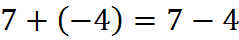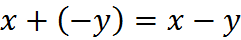Subtracting a negative number is the same as adding a positive number. For example,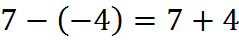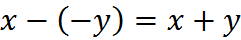## Multiplying and Dividing

Multiplying a negative number by a positive number, or a positive number by a negative number, makes the answer negative.

For example,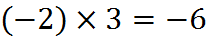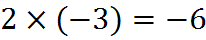It’s a good habit to write parentheses around negative numbers, as above. Doing so helps reduce preventable errors. Also, writing parentheses allows you to convey multiplication without using the multiplication symbol. It’s a good idea to omit the multiplication symbol, because in a handwritten context, it is too easily confused with the variable x. In this book, we will follow printed convention and often use the symbol, but you should make a habit of writing on your scratch paper (and ultimately your noteboard in the testing center) in this fashion: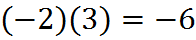and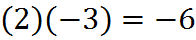Multiplying a negative number by a negative number makes the answer positive.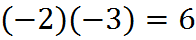You do the same for dividing.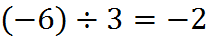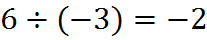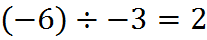## Exponentiation

Taking a negative number to an even power generates a positive number.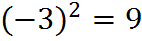In this case, writing the -3 in parentheses is required to communicate that we are squaring the negative number, not multiplying the squared number by -1.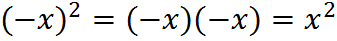Taking a negative number to an odd power generates a negative number every time.

For example,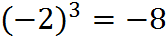or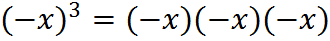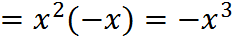The -1’s cancel out to become positive 1’s in pairs. When the exponent is odd, there’s always one of them left over, making the overall product negative.

## Order of Operations

As we saw above, parentheses are important when dealing with exponents of negative powers. We get a different result without parentheses,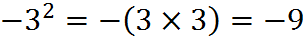…than we do with parentheses: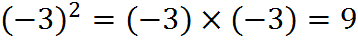This difference is explained by “order of operations,” which is covered in an upcoming chapter. In those terms, the exponent (“E” in PEMDAS) comes before multiplication by -1 (“M” in PEMDAS) unless we make the multiplication by -1 come first by putting it in parentheses (“P” in PEMDAS).

Welcome! You are encouraged to register with the site and login (for free). When you register, you support the site and your question history is saved.